I have written notes throughout this notebook to describe a little what I am doing since everything was done in R and not Python.

The important files are the following: "ets_notebook.ipynb", "submission.csv", "TSfeatures_train_val.csv", "TSfeatures_test.csv", "test.csv" and "train.csv".

• The "ets_notebook.ipynb" documents everything that I did so that you do not have to re-create / re-run everything.
• The "submission.csv" is the final submission - it contains a prediction probability and a binary variable of either "synthetic" or "real".
• The "TSfeatures_train_val.csv" consist of the data I created which is fed into the Machine Learning classifier (XGBoost).
• The "TSfeatures_test.csv" are the time series features fed to the out-of-sample test data.
• The "train.csv" and "test.csv" are the train and test dataset that ETS provided.

Part 1 of the notebook:

• Cleans the data and puts it into a better format for analysis.
• Simple plot of some returns for the Synthetic and Real financial time series.
• Box-plots of average returns and standard deviations.
• Computes the Durbin-Watson test statistics for both Synthetic and Real time series and box-plots.
• Plot the 10 day rolling mean and standard deviations for a random time series for Synthetic and real data.
• Dickey Fuller test on both the Synthetic and real time series.
• Jarque-Bera Test For Normality on the Synthetic and real time series.
• ACF Plots for both the Synthetic and real time series.

Part 2 of the notebook:

• Creates the time series features.
• Splits the train.csv into "train" and "validation" datasets.
• Puts the data into the correct format for XGBoost.
• Sets up and search over a parameter space to find the most optimal parameters for this dataset (on the train data).
• Outputs these parameters into a dataframe.
• Train the model using the optimal parameters found from the grid-search.
• Plot the feature importance scores - i.e. the most "important" variables that the model found when making its predictions.
• Assign a cut-off on the probability scores (> 0.5 then assign a 1 - real time series, <= 0.5 then assign a 0 for Synthetic).
• Compute the Confusion Matrix and analyse the 'in-sample' validation results.

Part 3 of the notebook:

• Create the "test.csv" feautres just as before and save as "TSfeatures_test.csv".
• Load in the "TSfeatures_train_val.csv" and "TSfeatures_test.csv" which were created from "train.csv" and "test.csv".
• Set up and run the XGBoost model using the optimal parameters found from the cross-validation grid search in "Part 2".
• Plot the predicted probability density plot as before in "Part 2".
• Set the cut-off threshold as the mean prediction score (0.465) which is close to the (0.500) score from "Part 2".
• Save the results as "submission.csv".
In :
list.files()

1. 'data.csv'
2. 'ets_model_3.R'
3. 'ETS_model.R'
4. 'ets_notebook.ipynb'
5. 'md5_hashes.docx'
6. 'reto_data_science.pdf'
7. 'sample_submission.csv'
8. 'submission.csv'
9. 'submission2.csv'
10. 'test.7z'
11. 'test.csv'
12. 'train.7z'
13. 'train.csv'
14. 'TSfeatures_test.csv'
15. 'TSfeatures_train_val.csv'
16. 'TSfeatures.csv'
In :
rm(list = ls())
options(scipen=999)
setwd('C:/Users/Matt/Desktop/Data Science Challenge')
shhh <- suppressPackageStartupMessages

shhh(library(dplyr))
library(TSrepr)
library(ggplot2)
library(data.table)
library(cluster)
library(clusterCrit)
library(fractalrock)
library(cowplot)
library(tidyr)
library(tidyquant)
library(lmtest)
library(aTSA)
library(tsoutliers)
library(tsfeatures)
library(xgboost)
library(caret)


Parsed with column specification:
cols(
.default = col_double()
)
See spec(...) for full column specifications.
Parsed with column specification:
cols(
.default = col_double()
)
See spec(...) for full column specifications.


# PART 1:¶

In :
######################################################################
################# Clean the data #####################################

# Since the "features" are daily time series, I just choose a random year and rename the feautres into more meaningful names
# Such as "2010-01-01", "2010-01-02", "2010-01-03" instead of "feature1", "feature2", "feature3" etc.
# Theres a "trading dates" package in R to get only the dates which are trading dates.
colnames(train_val) <- getTradingDates('2010-01-01', obs = 260)
colnames(train_val)[ncol(train_val)] <- "class"
colnames(test) <- getTradingDates('2010-01-01', obs = 260)
test$dataset <- "test" train_val$dataset <- "train"

In :
######################################################################
################# Rearrange the data #################################

# I melt the data for easier analysis, now the data is in a long format.

# "Class" corresponds to whether the asset is Synthetic or Real
# "Dataset" tells me where the data came from
# "row_id" - corresponds to a unique ID assigned to each asset both "(Synthetic & Real)"
# "Variable" is the column names of the original dataset (feature1, feature2, ... , featureN) converted to some date
# "Value" is the daily returns

df <- train_val %>%
mutate(row_id = row_number()) %>%
melt(., measure.vars = 1:260) %>%
arrange(row_id)

dim(df)

classdatasetrow_idvariablevalue
0 train 1 2009-01-05 0.006286455
0 train 1 2009-01-06 0.004407363
0 train 1 2009-01-07 -0.038088652
0 train 1 2009-01-08 0.025285012
0 train 1 2009-01-09 -0.006578773
0 train 1 2009-01-12 0.005713677
1. 3120000
2. 5
In :
# Plot some returns - I only plot a random sample of 20 assets for each Synthetic vs Real.

ret_plot0 <- df %>%
filter(class == 0) %>%
group_by(row_id) %>%
nest() %>%
sample_n(20) %>%
unnest() %>%
ggplot(aes(x = variable, y = value)) +
geom_line(aes(group = factor(row_id), color = factor(row_id))) +
ggtitle("Synthetic Financial Time Series") +
theme_classic() +
theme(axis.text.x = element_blank(), legend.position = "bottom", legend.title = element_blank())

ret_plot1 <- df %>%
filter(class == 1) %>%
group_by(row_id) %>%
nest() %>%
sample_n(20) %>%
unnest() %>%
ggplot(aes(x = variable, y = value)) +
geom_line(aes(group = factor(row_id), color = factor(row_id))) +
ggtitle("Real Financial Time Series") +
theme_classic() +
theme(axis.text.x = element_blank(), legend.position = "bottom", legend.title = element_blank())

plot_grid(ret_plot0, ret_plot1)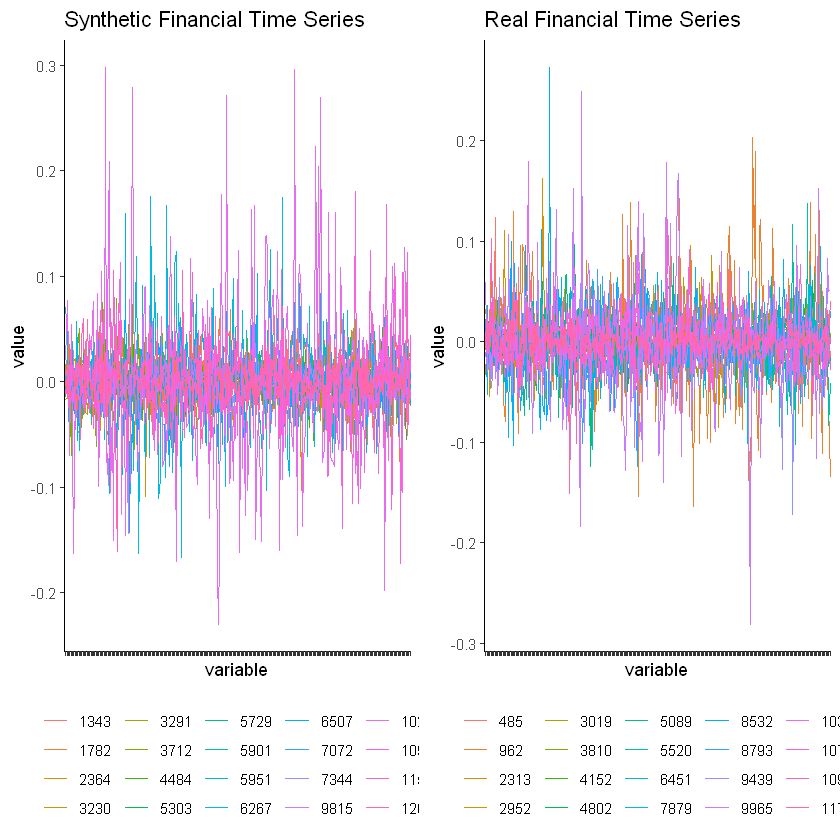In :
# I compute the average returns and standard deviations over the period for all asssets and compare each class.

ave_box <- df %>%
group_by(class, row_id) %>%
summarise(mean = mean(value)) %>%
ggplot(aes(x = factor(class), y = mean, color = factor(class))) +
geom_boxplot(show.legend = FALSE) +
ggtitle("Syn vs Real Average Returns") +
xlab("Class") +
ylab("Average Returns") +
theme_tq()

sd_box <- df %>%
group_by(class, row_id) %>%
summarise(sd = sd(value)) %>%
ggplot(aes(x = factor(class), y = sd, color = factor(class))) +
geom_boxplot(show.legend = FALSE) +
ggtitle("Syn vs Real Standard Deviations") +
xlab("Class") +
ylab("Standard Deviation") +
theme_tq()

plot_grid(ave_box, sd_box)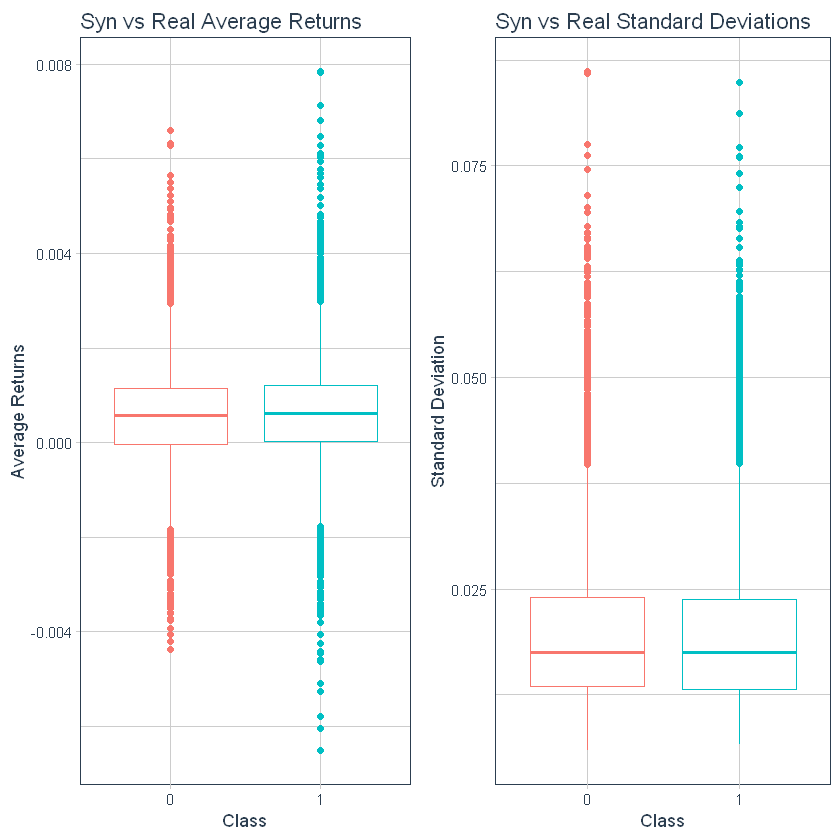In :
# I calculate the Durbin-Watson statistic and use the "tidy()" function to summarise the key information from the calculation.

dw_test_class_zero <- df %>%
dplyr::filter(class == 0) %>%
nest(-row_id) %>%
mutate(dw_res = map(data, ~ broom::tidy(lmtest::dwtest(value ~ 1, data = .x)))) %>%
unnest(dw_res) %>%
mutate(class = "0")

In :
# Here I do the exact same thing as above but this time for the class == 1 data.

dw_test_class_one <- df %>%
filter(class == 1) %>%
nest(-row_id) %>%
mutate(dw_res = map(data, ~ broom::tidy(lmtest::dwtest(value ~ 1, data = .x)))) %>%
unnest(dw_res) %>%
mutate(class = "1")

In :
# I bind the rows together and plot a box-plot.

rbind(dw_test_class_zero, dw_test_class_one) %>%
group_by(class) %>%
ggplot(aes(x = factor(class), y = statistic, color = factor(class))) +
geom_boxplot(show.legend = FALSE) +
ggtitle("Durbin Watson Box Plot Statistics") +
xlab("Class") +
ylab("Durbin Watson") +
theme_tq()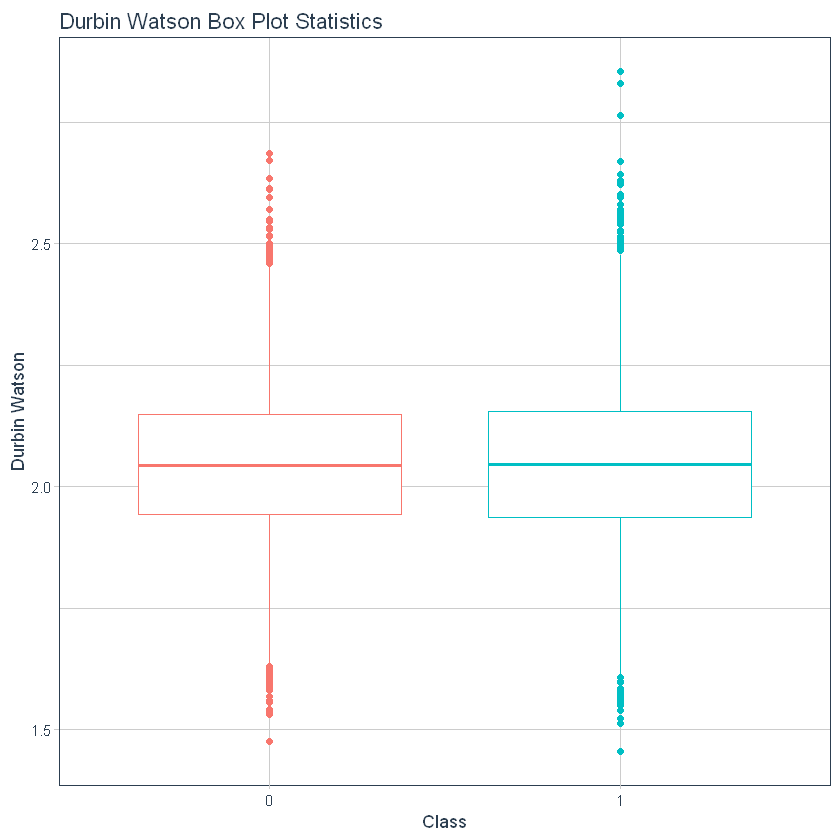In :
# Rolling mean and standard deviations
# I only use a random sample of 1 of each class of the grouped observations to save on memory and to make the plot more readable.
# The rollowing window is 10 days
# I use the tq_mutate functionality from the "tidyquant" package to keep things in a "tidy" format as per the "tidyverse" 'rules'.
# In the plot "value" is the returns, "mean_10" is the 10 day rolling mean and "sd_10" is the 10 day rolling standard deviation.

plot0 <- df %>%
filter(class == 0) %>%
as_tibble() %>%
group_by(row_id) %>%
nest() %>%
sample_n(1) %>%
unnest() %>%
mutate(variable = as.Date(variable)) %>%
tq_mutate(
select     = value,
mutate_fun = rollapply,
width      = 10,
align      = "right",
FUN        = mean,
na.rm      = TRUE,
col_rename = "mean_10"
) %>%
tq_mutate(
select     = value,
mutate_fun = rollapply,
width      = 10,
align      = "right",
FUN        = sd,
na.rm      = TRUE,
col_rename = "sd_10"
) %>%
melt(measure.vars = 5:7) %>%
setNames(c("row_id", "class", "dataset", "date", "variable", "value")) %>%
ggplot(aes(x = date)) +
geom_line(aes(y = value, colour = variable)) +
ggtitle("Synthetic Financial Time Series Rolling Mean and Standard Deviation") +
theme_classic() +
scale_colour_manual(values = c("#1f77b4", "red", "black")) +
theme(axis.text.x = element_blank(), legend.position = "bottom", legend.title = element_blank())

plot1 <- df %>%
filter(class == 1) %>%
as_tibble() %>%
group_by(row_id) %>%
nest() %>%
sample_n(1) %>%
unnest() %>%
mutate(variable = as.Date(variable)) %>%
tq_mutate(
select     = value,
mutate_fun = rollapply,
width      = 10,
align      = "right",
FUN        = mean,
na.rm      = TRUE,
col_rename = "mean_10"
) %>%
tq_mutate(
select     = value,
mutate_fun = rollapply,
width      = 10,
align      = "right",
FUN        = sd,
na.rm      = TRUE,
col_rename = "sd_10"
) %>%
melt(measure.vars = 5:7) %>%
setNames(c("row_id", "class", "dataset", "date", "variable", "value")) %>%
ggplot(aes(x = date)) +
geom_line(aes(y = value, colour = variable)) +
ggtitle("Real Financial Time Series Rolling Mean and Standard Deviation") +
theme_classic() +
scale_colour_manual(values = c("#1f77b4", "red", "black")) +
theme(axis.text.x = element_blank(), legend.position = "bottom", legend.title = element_blank())

plot_grid(plot0, plot1)

Warning message:
“Removed 18 rows containing missing values (geom_path).”Warning message:
“Removed 18 rows containing missing values (geom_path).”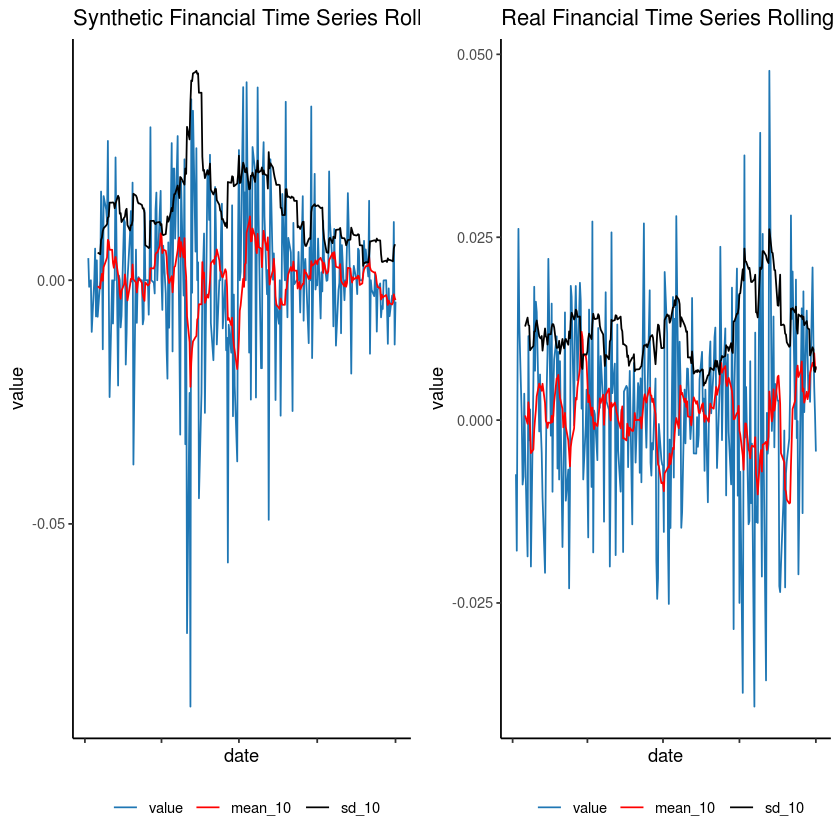In :
# Dickey Fuller test on the 0 class
# I only randomly sample 1 of the assets for the 0 class to save on output space

df %>%
filter(class == 0) %>%
group_by(row_id) %>%
nest() %>%
sample_n(1) %>%
unnest() %>%
nest(-row_id) %>%
mutate(adf_res = map(data, ~ adf.test(.x$value))) %>% unnest(adf_res)  Augmented Dickey-Fuller Test alternative: stationary Type 1: no drift no trend lag ADF p.value [1,] 0 -17.11 0.01 [2,] 1 -11.01 0.01 [3,] 2 -9.47 0.01 [4,] 3 -9.09 0.01 [5,] 4 -8.61 0.01 Type 2: with drift no trend lag ADF p.value [1,] 0 -17.13 0.01 [2,] 1 -11.03 0.01 [3,] 2 -9.50 0.01 [4,] 3 -9.16 0.01 [5,] 4 -8.67 0.01 Type 3: with drift and trend lag ADF p.value [1,] 0 -17.16 0.01 [2,] 1 -11.08 0.01 [3,] 2 -9.55 0.01 [4,] 3 -9.19 0.01 [5,] 4 -8.78 0.01 ---- Note: in fact, p.value = 0.01 means p.value <= 0.01  A tibble: 3 × 2 row_idadf_res <int><list> 67420.000000, 1.000000, 2.000000, 3.000000, 4.000000, -17.114508, -11.013833, -9.465615, -9.085421, -8.609105, 0.010000, 0.010000, 0.010000, 0.010000, 0.010000 67420.000000, 1.000000, 2.000000, 3.000000, 4.000000, -17.128116, -11.033299, -9.504570, -9.157584, -8.669078, 0.010000, 0.010000, 0.010000, 0.010000, 0.010000 67420.000000, 1.000000, 2.000000, 3.000000, 4.000000, -17.156757, -11.081321, -9.545201, -9.185512, -8.780369, 0.010000, 0.010000, 0.010000, 0.010000, 0.010000 In : # Dickey Fuller test on the 1 class # I only randomly sample 1 of the assets for the 1 class to save on output space df %>% filter(class == 1) %>% group_by(row_id) %>% nest() %>% sample_n(1) %>% unnest() %>% nest(-row_id) %>% mutate(adf_res = map(data, ~ adf.test(.x$value))) %>%

Augmented Dickey-Fuller Test
alternative: stationary

Type 1: no drift no trend
[1,]   0 -16.37    0.01
[2,]   1 -12.03    0.01
[3,]   2 -10.19    0.01
[4,]   3  -9.02    0.01
[5,]   4  -7.52    0.01
Type 2: with drift no trend
[1,]   0 -16.50    0.01
[2,]   1 -12.22    0.01
[3,]   2 -10.41    0.01
[4,]   3  -9.29    0.01
[5,]   4  -7.82    0.01
Type 3: with drift and trend
[1,]   0 -16.47    0.01
[2,]   1 -12.19    0.01
[3,]   2 -10.39    0.01
[4,]   3  -9.27    0.01
[5,]   4  -7.80    0.01
----
Note: in fact, p.value = 0.01 means p.value <= 0.01

A tibble: 3 × 2
<int><list>
110850.000000, 1.000000, 2.000000, 3.000000, 4.000000, -16.371976, -12.029433, -10.186513, -9.017429, -7.522447, 0.010000, 0.010000, 0.010000, 0.010000, 0.010000
110850.000000, 1.000000, 2.000000, 3.000000, 4.000000, -16.501759, -12.215099, -10.411252, -9.290359, -7.819255, 0.010000, 0.010000, 0.010000, 0.010000, 0.010000
110850.000000, 1.000000, 2.000000, 3.000000, 4.000000, -16.469330, -12.190427, -10.391839, -9.271585, -7.801521, 0.010000, 0.010000, 0.010000, 0.010000, 0.010000
In :
##################### Jarque-Bera Test For Normality #####

# For both classes I take a random sample of 1 observation from each class (Synthetic and Real financial series)

jb_zero <- df %>%
filter(class == 0) %>%
group_by(row_id) %>%
nest() %>%
sample_n(1) %>%
unnest() %>%
nest(-row_id) %>%
mutate(JarqueBeraTest = map(data, ~ JarqueBera.test(.x$value))) print("Jarque-Bera Test on the 0 - Synthetic class") jb_zero$JarqueBeraTest

jb_one <- df %>%
filter(class == 0) %>%
group_by(row_id) %>%
nest() %>%
sample_n(1) %>%
unnest() %>%
nest(-row_id) %>%
mutate(JarqueBeraTest = map(data, ~ JarqueBera.test(.x$value))) print("Jarque-Bera Test on the 1 - Real class") jb_one$JarqueBeraTest

 "Jarque-Bera Test on the 0 - Synthetic class"

[]

Jarque Bera Test

data:  .x$value X-squared = 3.2039, df = 2, p-value = 0.2015 Skewness data: .x$value
statistic = 0.065126, p-value = 0.6681

Kurtosis

data:  .x$value statistic = 3.528, p-value = 0.08224   "Jarque-Bera Test on the 1 - Real class"  [] Jarque Bera Test data: .x$value
X-squared = 85.004, df = 2, p-value < 0.00000000000000022

Skewness

data:  .x$value statistic = 0.021118, p-value = 0.8894 Kurtosis data: .x$value
statistic = 5.8008, p-value < 0.00000000000000022


In :
######################################################################
################# ACF plots ##########################################

# I only use 4 observations for these plots, 2 from the "synthetic" class and 2 from the "real" class.

df %>%
filter(row_id == 6422 | row_id == 8967 | row_id == 6080 | row_id == 	5734) %>%
mutate(date = as.Date(variable)) %>%
ggplot(aes(x = date)) +
geom_line(aes(y = value), color = "red", alpha = 0.4) +
geom_hline(yintercept = 0) +
facet_wrap(~ row_id + class) +
theme_tq()

acf_data <- df %>%
dplyr::filter(row_id == 6422 | row_id == 8967 | row_id == 6080 | row_id == 	5734) %>%
mutate(date = as.Date(variable))

df_acf <- acf_data %>%
group_by(row_id) %>%
summarise(list_acf = list(acf(value, plot=FALSE))) %>%
mutate(acf_vals = purrr::map(list_acf, ~as.numeric(.x$acf))) %>% select(-list_acf) %>% unnest() %>% group_by(row_id) %>% mutate(lag = row_number() - 1) df_ci <- acf_data %>% group_by(row_id) %>% summarise(ci = qnorm((1 + 0.95)/2)/sqrt(n())) ggplot(df_acf, aes(x = lag, y = acf_vals)) + geom_bar(stat="identity", width=.05) + geom_hline(yintercept = 0) + geom_hline(data = df_ci, aes(yintercept = -ci), color="blue", linetype="dotted") + geom_hline(data = df_ci, aes(yintercept = ci), color="blue", linetype="dotted") + labs(x="Lag", y="ACF") + facet_wrap(~ row_id) + theme_tq()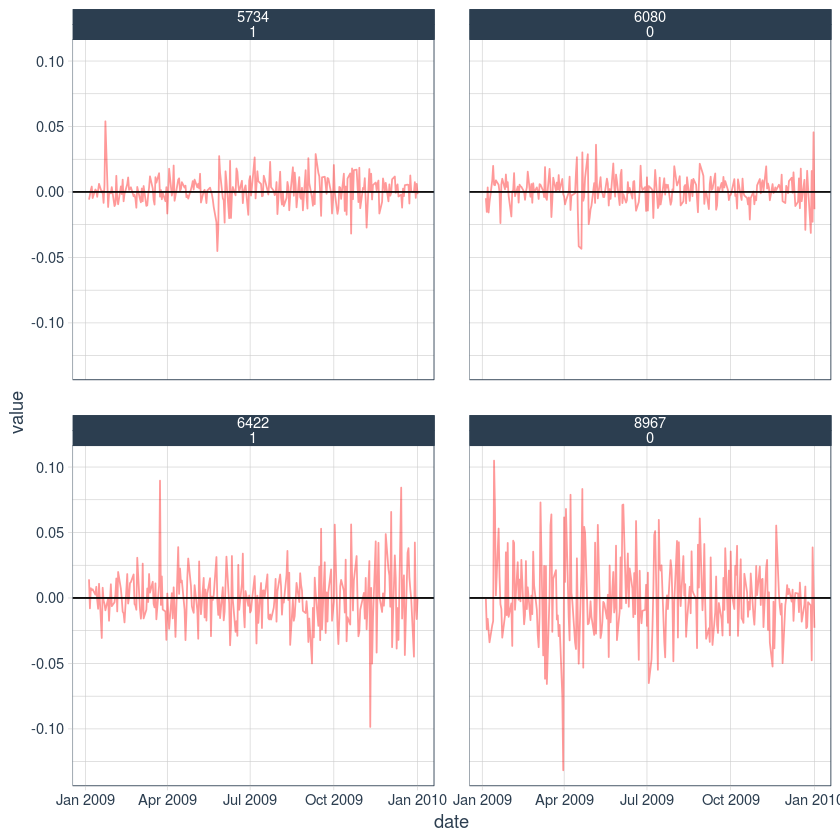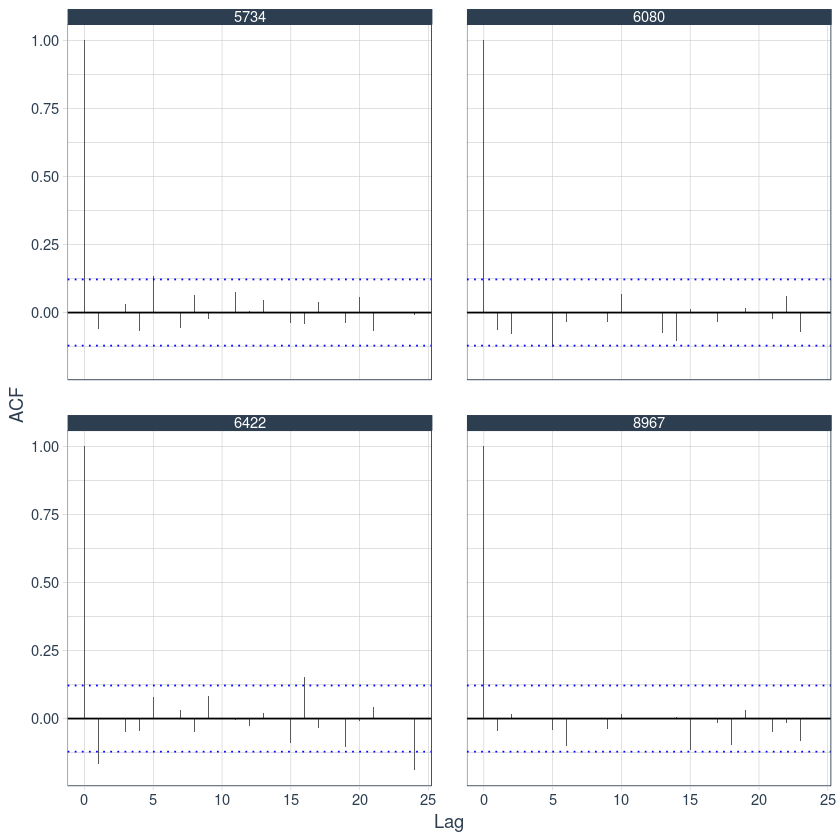# ------- END OF DATA ANALYSIS --------¶ # PART 2:¶ In : ###################################################################### ################# Generate Time Series Features ###################### # I create some time series features from the package "tsfeatures". There are 40+ functions in the "tsfeatures" package # which can generate approximately 106 time series features. # Due to memory issues I am only able to create a few of the features, therefore I randomly sample 10 features from the # "tsfeatures" package. We could also add in technical indicators from the "PerformanceAnalytics" or "TTR" packages (I omit these # here, however creating 'functions2 <- ls("package:TTR")' and adding it to the 'summarise' command will work.) functions <- ls("package:tsfeatures")[1:42] # functions <- sample(functions, 20) Stats <- df %>% group_by(row_id, class) %>% # nest() %>% # sample_n(5) %>% # unnest() %>% nest(-row_id, -class) %>% group_by(row_id, class) %T>% {options(warn = -1)} %>% summarise(Statistics = map(data, ~ data.frame( bind_cols( tsfeatures(.x$value, functions))))) %>%
unnest(Statistics)

#print("Generated 106 Time Series features")

# A tibble: 12,000 x 51
# Groups:   row_id [12,000]
row_id class trev_num_trev_n… unitroot_pp flat_spots embed2_incircle
<int> <dbl>            <dbl>       <dbl>      <dbl>           <dbl>
1      1     0           0.121        -250.          4           0.393
2      2     0          -0.477        -256.          4           0.429
3      3     1          -0.876        -258.          6           0.467
4      4     0          -0.994        -262.          7           0.456
5      5     0          -0.717        -324.          9           0.656
6      6     0           0.0899       -262.          5           0.435
7      7     1           0.0132       -254.          7           0.525
8      8     1           0.167        -243.          6           0.399
9      9     0           0.288        -234.          5           0.578
10     10     0          -0.115        -246.         10           0.585
# … with 11,990 more rows, and 45 more variables: max_level_shift <dbl>,
#   time_level_shift <dbl>, autocorr_features_embed2_incircle_1 <dbl>,
#   autocorr_features_embed2_incircle_2 <dbl>, autocorr_features_ac_9 <dbl>,
#   autocorr_features_firstmin_ac <dbl>, autocorr_features_trev_num <dbl>,
#   autocorr_features_motiftwo_entro3 <dbl>,
#   autocorr_features_walker_propcross <dbl>, std1st_der <dbl>,
#   lumpiness <dbl>, arch_acf <dbl>, garch_acf <dbl>, arch_r2 <dbl>,
#   garch_r2 <dbl>, dist_features_histogram_mode_10 <dbl>,
#   dist_features_outlierinclude_mdrmd <dbl>, unitroot_kpss <dbl>,
#   spreadrandomlocal_meantaul <dbl>, alpha <dbl>, beta <dbl>,
#   crossing_points <dbl>, frequency <dbl>, nperiods <dbl>,
#   seasonal_period <dbl>, trend <dbl>, spike <dbl>, linearity <dbl>,
#   curvature <dbl>, e_acf1 <dbl>, e_acf10 <dbl>, entropy <dbl>, x_acf1 <dbl>,
#   x_acf10 <dbl>, diff1_acf1 <dbl>, diff1_acf10 <dbl>, diff2_acf1 <dbl>,
#   diff2_acf10 <dbl>, outlierinclude_mdrmd_outlierinclude_mdrmd <dbl>,
#   fluctanal_prop_r1_fluctanal_prop_r1 <dbl>, hurst <dbl>, x_pacf5 <dbl>,
#   diff1x_pacf5 <dbl>, diff2x_pacf5 <dbl>,
#   scal_features_fluctanal_prop_r1 <dbl>

In [ ]:
#write.csv(Stats, "TSfeatures_train_val.csv")

In :
Stats <- read.csv("TSfeatures_train_val.csv")
list.files()

1. 'data.csv'
2. 'ETS_model.R'
3. 'ets_model_3.R'
4. 'ets_notebook.ipynb'
5. 'md5_hashes.docx'
6. 'reto_data_science.pdf'
7. 'sample_submission.csv'
8. 'submission.csv'
9. 'submission2.csv'
10. 'test.7z'
11. 'test.csv'
12. 'train.7z'
13. 'train.csv'
14. 'TSfeatures.csv'
15. 'TSfeatures_test.csv'
16. 'TSfeatures_train_val.csv'
Xrow_idclassac_9_ac_9acf_features_x_acf1acf_features_x_acf10acf_features_diff1_acf1acf_features_diff1_acf10acf_features_diff2_acf1acf_features_diff2_acf10...tsfeatures_entropytsfeatures_x_acf1tsfeatures_x_acf10tsfeatures_diff1_acf1tsfeatures_diff1_acf10tsfeatures_diff2_acf1tsfeatures_diff2_acf10unitroot_kpssunitroot_ppwalker_propcross
1 1 0 -0.067527460 0.0097094450.05268970 -0.5005299 0.3297018 -0.6772403 0.6124739 ... 0.9840151 0.0097094450.05268970 -0.5005299 0.3297018 -0.6772403 0.6124739 0.09938291 -249.7732 0.5405405
2 2 0 -0.042157717-0.0075901950.03874814 -0.5171529 0.3129147 -0.6727897 0.5379301 ... 0.9864332 -0.0075901950.03874814 -0.5171529 0.3129147 -0.6727897 0.5379301 0.04145992 -256.0485 0.5019305
3 3 1 0.009959832-0.0405928740.04490357 -0.5026683 0.3471209 -0.6718885 0.6109006 ... 0.9868568 -0.0405928740.04490357 -0.5026683 0.3471209 -0.6718885 0.6109006 0.07756983 -258.1295 0.5328185
4 4 0 -0.042874797-0.0443618930.06158667 -0.4571442 0.3184053 -0.5906478 0.4361178 ... 0.9790521 -0.0443618930.06158667 -0.4571442 0.3184053 -0.5906478 0.4361178 0.21296332 -262.0781 0.4903475
5 5 0 0.025931211-0.2447834960.14691301 -0.5810073 0.4796508 -0.6799229 0.6232529 ... 0.9723766 -0.2447834960.14691301 -0.5810073 0.4796508 -0.6799229 0.6232529 0.15063439 -323.5672 0.5289575
6 6 0 -0.076116613 0.0468555780.08583475 -0.5253131 0.3438031 -0.6901570 0.6130725 ... 0.9806218 0.0468555780.08583475 -0.5253131 0.3438031 -0.6901570 0.6130725 0.02594138 -262.3484 0.5250965
In :
######################################################################
################# Train and XGBoost model on the TS Features #########

#Stats <- Stats %>%
#  select_if(~sum(!is.na(.)) > 0)

# Split the training set up between train and a small validation set
smp_size <- floor(0.75 * nrow(Stats))
#set.seed(123)
train_ind <- sample(seq_len(nrow(Stats)), size = smp_size)

train <- Stats[train_ind, ]
val <- Stats[-train_ind, ]

In :
# We have 106 time series features for the model to learn from.

x_train <- train %>%
ungroup() %>%
select(-class, -row_id, -X) %>%
as.matrix()

x_val <- val %>%
ungroup() %>%
select(-class, -row_id, -X) %>%
as.matrix()

y_train <- train %>%
ungroup() %>%
pull(class)

y_val <- val %>%
ungroup() %>%
pull(class)

print("How does the X variables look like?")
x_train %>%
as_tibble() %>%

print("How does the Y variables look like?")
y_train %>%
as_tibble() %>%

 "How does the X variables look like?"

ac_9_ac_9acf_features_x_acf1acf_features_x_acf10acf_features_diff1_acf1acf_features_diff1_acf10acf_features_diff2_acf1acf_features_diff2_acf10ARCH.LMautocorr_features_embed2_incircle_1autocorr_features_embed2_incircle_2...tsfeatures_entropytsfeatures_x_acf1tsfeatures_x_acf10tsfeatures_diff1_acf1tsfeatures_diff1_acf10tsfeatures_diff2_acf1tsfeatures_diff2_acf10unitroot_kpssunitroot_ppwalker_propcross
0.023236441-0.0746509710.02038917 -0.5603844 0.3214731 -0.6947503 0.5228883 0.02645647 0.4749035 0.6949807 ... 0.9907267 -0.0746509710.02038917 -0.5603844 0.3214731 -0.6947503 0.5228883 0.08418223 -295.5967 0.5637066
0.089101393-0.0051550640.13301470 -0.4885461 0.4353276 -0.6773534 0.7858669 0.22605361 0.5907336 0.7451737 ... 0.9636886 -0.0051550640.13301470 -0.4885461 0.4353276 -0.6773534 0.7858669 0.07434871 -225.7176 0.4903475
-0.015845671 0.0452321470.02809074 -0.4489214 0.2210296 -0.6291573 0.4179265 0.01533997 0.5658915 0.7674419 ... 0.9918732 0.0452321470.02809074 -0.4489214 0.2210296 -0.6291573 0.4179265 0.05921490 -227.0271 0.4633205
-0.040078185 0.0242022030.06762123 -0.4316614 0.3067859 -0.6202015 0.5529502 0.09864609 0.5891473 0.7674419 ... 0.9804658 0.0242022030.06762123 -0.4316614 0.3067859 -0.6202015 0.5529502 0.09948075 -227.6157 0.5057915
-0.007340002-0.1728313000.04932286 -0.5434486 0.3170055 -0.6880143 0.5446340 0.02723756 0.3976834 0.6525097 ... 0.9837865 -0.1728313000.04932286 -0.5434486 0.3170055 -0.6880143 0.5446340 0.07212473 -274.1723 0.5482625
-0.024278095 0.0929821930.02774284 -0.4618626 0.2562180 -0.6418039 0.4921543 0.11114889 0.5234375 0.6757812 ... 0.9831277 0.0929821930.02774284 -0.4618626 0.2562180 -0.6418039 0.4921543 0.04312335 -232.2476 0.4671815
 "How does the Y variables look like?"

value
0
0
0
1
0
1
In :
# XGBoost expects a 'special' type of matrix
dtrain <- xgb.DMatrix(data = as.matrix(x_train), label = y_train, missing = "NaN")
dval <- xgb.DMatrix(data = as.matrix(x_val), label = y_val, missing = "NaN")

In :
######################################################################
################# XGBoost Grid Search to locate Optimal Parameters ###

##############################################################################################################################
# NOTE: This section was taken from the first chapter of my PhD where I needed to search over a parameter space to locate the
# most optimal parameters - I have just adapted it for this problem of Time Series Classification.
# Its simple enough to add parameters and different values - I just optimise a few important parameters from domain knowledge
# of the XGBoost model for this task, i.e depth and eta are quite important in gradient boosting.

# 1) I create a "grid" with different parameter values or combinations of parameter values
# 2) I apply cross validation over the parameter space to fine the most optimal values for the XGBoost model.
# 3) I print the model parameters which give the best train / (in-sample test) results in a data table.
##############################################################################################################################

# Grid Search Parameters:
# 1)
searchGridSubCol <- expand.grid(subsample = c(1), #Range (0,1], default = 1, set to 0.5 will prevent overfitting
colsample_bytree = c(1), #Range (0,1], default = 1
max_depth = c(5, 8, 14), #Range (0, inf], default = 6
min_child = c(1), #Range (0, inf], default = 1
eta = c(0.1, 0.05, 0.3), #Range (0,1], default = 0.3
gamma = c(0), #Range (0, inf], default = 0
lambda = c(1), #Default = 1, L2 regularisation on weights, higher the more conservative the model
alpha = c(0), #Default = 0, L1 regularisation on weights, higher the more conservative the model
max_delta_step = c(0), #Range (0, inf], default = 0 (Helpful for logisitc regression when class is extremely imbalanced, set to value 1-10 may help control the update)
colsample_bylevel = c(1) #Range (0,1], default = 1
)

ntrees = 200
nfold <- 10
watchlist <- list(train = dtrain, test = dval)

# 2)
system.time(
AUCHyperparameters <- apply(searchGridSubCol, 1, function(parameterList){
#Extract Parameters to test
currentSubsampleRate <- parameterList[["subsample"]]
currentColsampleRate <- parameterList[["colsample_bytree"]]
currentDepth <- parameterList[["max_depth"]]
currentEta <- parameterList[["eta"]]
currentMinChild <- parameterList[["min_child"]]
gamma <- parameterList[["gamma"]]
lambda <- parameterList[["lambda"]]
alpha <- parameterList[["alpha"]]
max_delta_step <- parameterList[["max_delta_step"]]
colsample_bylevel <- parameterList[["colsample_bylevel"]]
xgboostModelCV <- xgb.cv(data =  dtrain,
nrounds = ntrees,
nfold = nfold,
showsd = TRUE,
metrics = c("auc", "logloss", "error"),
verbose = TRUE,
"eval_metric" = c("auc", "logloss", "error"),
"objective" = "binary:logistic", #Outputs a probability "binary:logitraw" - outputs score before logistic transformation
"max.depth" = currentDepth,
"eta" = currentEta,
"gamma" = gamma,
"lambda" = lambda,
"alpha" = alpha,
"subsample" = currentSubsampleRate,
"colsample_bytree" = currentColsampleRate,
print_every_n = 50, # print ever 50 trees to reduce the outputs printed.
"min_child_weight" = currentMinChild,
booster = "gbtree", #booster = "dart"  #using dart can help improve accuracy.
early_stopping_rounds = 10,
watchlist = watchlist,
seed = 1234)
xvalidationScores <<- as.data.frame(xgboostModelCV$evaluation_log) train_auc_mean <- tail(xvalidationScores$train_auc_mean, 1)
test_auc_mean <- tail(xvalidationScores$test_auc_mean, 1) train_logloss_mean <- tail(xvalidationScores$train_logloss_mean, 1)
test_logloss_mean <- tail(xvalidationScores$test_logloss_mean, 1) train_error_mean <- tail(xvalidationScores$train_error_mean, 1)
test_error_mean <- tail(xvalidationScores$test_error_mean, 1) output <- return(c(train_auc_mean, test_auc_mean, train_logloss_mean, test_logloss_mean, train_error_mean, test_error_mean, xvalidationScores, currentSubsampleRate, currentColsampleRate, currentDepth, currentEta, gamma, lambda, alpha, max_delta_step, colsample_bylevel, currentMinChild)) hypemeans <- which.max(AUCHyperparameters[]$test_auc_mean)
output2 <- return(hypemeans)
}))

	train-auc:0.709444+0.005449	train-logloss:0.679352+0.000266	train-error:0.356012+0.007934	test-auc:0.674091+0.017564	test-logloss:0.681697+0.001146	test-error:0.380111+0.011477
Multiple eval metrics are present. Will use test_error for early stopping.
Will train until test_error hasn't improved in 10 rounds.

	train-auc:0.864482+0.003830	train-logloss:0.509142+0.003445	train-error:0.223012+0.004731	test-auc:0.735318+0.012974	test-logloss:0.599101+0.009049	test-error:0.336111+0.013809
Stopping. Best iteration:
	train-auc:0.886833+0.004311	train-logloss:0.486189+0.004434	train-error:0.199753+0.005421	test-auc:0.738559+0.013404	test-logloss:0.596390+0.009818	test-error:0.331111+0.013690

	train-auc:0.783311+0.005215	train-logloss:0.670555+0.000708	train-error:0.302667+0.004773	test-auc:0.660515+0.017584	test-logloss:0.680860+0.001373	test-error:0.387444+0.023878
Multiple eval metrics are present. Will use test_error for early stopping.
Will train until test_error hasn't improved in 10 rounds.

	train-auc:0.987024+0.002600	train-logloss:0.341604+0.007166	train-error:0.058951+0.006592	test-auc:0.743563+0.018166	test-logloss:0.593442+0.014256	test-error:0.328778+0.020985
Stopping. Best iteration:
	train-auc:0.983222+0.003323	train-logloss:0.356999+0.008188	train-error:0.069271+0.008111	test-auc:0.742024+0.017524	test-logloss:0.594408+0.013484	test-error:0.328111+0.021181

	train-auc:0.934010+0.008853	train-logloss:0.644586+0.002349	train-error:0.147864+0.013444	test-auc:0.643095+0.021261	test-logloss:0.679845+0.002381	test-error:0.408444+0.019696
Multiple eval metrics are present. Will use test_error for early stopping.
Will train until test_error hasn't improved in 10 rounds.

	train-auc:1.000000+0.000000	train-logloss:0.107373+0.004389	train-error:0.000000+0.000000	test-auc:0.754090+0.018546	test-logloss:0.595836+0.022702	test-error:0.318889+0.014783
Stopping. Best iteration:
	train-auc:1.000000+0.000000	train-logloss:0.077801+0.003230	train-error:0.000000+0.000000	test-auc:0.757667+0.018102	test-logloss:0.599993+0.024362	test-error:0.314111+0.013690

	train-auc:0.703941+0.002905	train-logloss:0.686155+0.000119	train-error:0.362555+0.004303	test-auc:0.665895+0.014237	test-logloss:0.687514+0.000620	test-error:0.383555+0.010655
Multiple eval metrics are present. Will use test_error for early stopping.
Will train until test_error hasn't improved in 10 rounds.

	train-auc:0.821497+0.001737	train-logloss:0.554493+0.001649	train-error:0.266444+0.003673	test-auc:0.729046+0.016835	test-logloss:0.608759+0.009447	test-error:0.338333+0.014633
Stopping. Best iteration:
	train-auc:0.840083+0.001913	train-logloss:0.535131+0.001830	train-error:0.248679+0.004101	test-auc:0.732550+0.017349	test-logloss:0.603786+0.010547	test-error:0.334556+0.014677

	train-auc:0.786423+0.009774	train-logloss:0.681408+0.000625	train-error:0.300975+0.010759	test-auc:0.662952+0.016292	test-logloss:0.686516+0.000730	test-error:0.387111+0.009339
Multiple eval metrics are present. Will use test_error for early stopping.
Will train until test_error hasn't improved in 10 rounds.

Stopping. Best iteration:
	train-auc:0.921793+0.006983	train-logloss:0.501812+0.004283	train-error:0.166691+0.010027	test-auc:0.725392+0.013456	test-logloss:0.613664+0.007937	test-error:0.338778+0.015615

	train-auc:0.930805+0.007333	train-logloss:0.668693+0.001006	train-error:0.151123+0.012435	test-auc:0.653053+0.022793	test-logloss:0.685441+0.001372	test-error:0.397889+0.019337
Multiple eval metrics are present. Will use test_error for early stopping.
Will train until test_error hasn't improved in 10 rounds.

	train-auc:1.000000+0.000000	train-logloss:0.208367+0.004190	train-error:0.000197+0.000158	test-auc:0.748054+0.016423	test-logloss:0.592041+0.015400	test-error:0.323667+0.014238
Stopping. Best iteration:
	train-auc:1.000000+0.000000	train-logloss:0.198295+0.003931	train-error:0.000136+0.000151	test-auc:0.749679+0.016039	test-logloss:0.591085+0.015690	test-error:0.320556+0.011069

	train-auc:0.706879+0.006937	train-logloss:0.656097+0.000996	train-error:0.358358+0.006934	test-auc:0.664376+0.014192	test-logloss:0.664288+0.002779	test-error:0.386222+0.015016
Multiple eval metrics are present. Will use test_error for early stopping.
Will train until test_error hasn't improved in 10 rounds.

Stopping. Best iteration:
	train-auc:0.856229+0.004436	train-logloss:0.507505+0.004026	train-error:0.231247+0.005317	test-auc:0.723719+0.016158	test-logloss:0.606779+0.011660	test-error:0.342444+0.014227

	train-auc:0.787977+0.005708	train-logloss:0.630568+0.002147	train-error:0.296852+0.006258	test-auc:0.670012+0.015879	test-logloss:0.660021+0.003552	test-error:0.385333+0.011757
Multiple eval metrics are present. Will use test_error for early stopping.
Will train until test_error hasn't improved in 10 rounds.

	train-auc:0.999944+0.000023	train-logloss:0.147026+0.005866	train-error:0.002593+0.000694	test-auc:0.737500+0.013444	test-logloss:0.626521+0.018879	test-error:0.329111+0.010539
Stopping. Best iteration:
	train-auc:0.999998+0.000003	train-logloss:0.106048+0.006895	train-error:0.000370+0.000322	test-auc:0.739784+0.012617	test-logloss:0.635691+0.018997	test-error:0.326889+0.013534

	train-auc:0.930660+0.010723	train-logloss:0.561804+0.007616	train-error:0.149531+0.015951	test-auc:0.653138+0.011059	test-logloss:0.662209+0.003700	test-error:0.398333+0.011224
Multiple eval metrics are present. Will use test_error for early stopping.
Will train until test_error hasn't improved in 10 rounds.

	train-auc:1.000000+0.000000	train-logloss:0.024778+0.000901	train-error:0.000000+0.000000	test-auc:0.751464+0.015141	test-logloss:0.673285+0.031905	test-error:0.318889+0.011320
Stopping. Best iteration:
	train-auc:1.000000+0.000000	train-logloss:0.026861+0.001092	train-error:0.000000+0.000000	test-auc:0.751326+0.014651	test-logloss:0.669634+0.030983	test-error:0.315111+0.010979


   user  system elapsed
1939.63   51.14  654.69 
In :
# 3)
output <- as.data.frame(t(sapply(AUCHyperparameters, '[', c(1:6, 20:29))))
varnames <- c("TrainAUC", "TestAUC", "TrainLogloss", "TestLogloss", "TrainError", "TestError", "SubSampRate", "ColSampRate", "Depth", "eta", "gamma", "lambda", "alpha", "max_delta_step", "col_sample_bylevel", "currentMinChild")
colnames(output) <- varnames
data.table(output)

TrainAUCTestAUCTrainLoglossTestLoglossTrainErrorTestErrorSubSampRateColSampRateDepthetagammalambdaalphamax_delta_stepcol_sample_bylevelcurrentMinChild
0.89772540.73901320.47426360.59542820.18780260.33300011 1 5 0.1 0 1 0 0 1 1
0.98906970.743927 0.33192950.59328340.05362960.32933331 1 8 0.1 0 1 0 0 1 1
1 0.75841380.06623220.60392680 0.31622231 1 14 0.1 0 1 0 0 1 1
0.84933960.73367570.52632020.60218690.239679 0.33833331 1 5 0.05 0 1 0 0 1 1
0.94307870.73226550.46426750.60551510.13776530.33966681 1 8 0.05 0 1 0 0 1 1
1 0.75182960.16986820.59013760 0.32188881 1 14 0.05 0 1 0 0 1 1
0.89324110.72392990.466469 0.60636630.19254310.34644451 1 5 0.3 0 1 0 0 1 1
1 0.74075830.08689160.64236660.00007380.32988891 1 8 0.3 0 1 0 0 1 1
1 0.75200430.02125220.68146350 0.31766671 1 14 0.3 0 1 0 0 1 1

# Optimal Parameters:¶

ntrees = 95,

eta = 0.1,

max_depth = 5,

With the other parameters left to default settings for simplicity.

Lets plug them into the XGB model

In :
#################################################################################
################# XGBoost Optimal Parameters from Cross Validation ##############

# This is the final training model where I use the most optimal parameters found over the grid space and plug them in here.

watchlist <- list("train" = dtrain)

params <- list("eta" = 0.1, "max_depth" = 5, "colsample_bytree" = 1, "min_child_weight" = 1, "subsample"= 1,
"objective"="binary:logistic", "gamma" = 1, "lambda" = 1, "alpha" = 0, "max_delta_step" = 0,
"colsample_bylevel" = 1, "eval_metric"= "auc",
"set.seed" = 176)

nround <- 95

In :
# Train the XGBoost model

xgb.model <- xgb.train(params, dtrain, nround, watchlist)
# Note: Plot AUC on for the in-sample train / validation scores

	train-auc:0.705973
	train-auc:0.719498
	train-auc:0.734230
	train-auc:0.740069
	train-auc:0.745137
	train-auc:0.746840
	train-auc:0.754894
	train-auc:0.759521
	train-auc:0.761794
	train-auc:0.764262
	train-auc:0.771451
	train-auc:0.773591
	train-auc:0.778484
	train-auc:0.781871
	train-auc:0.784020
	train-auc:0.784618
	train-auc:0.786377
	train-auc:0.789053
	train-auc:0.794144
	train-auc:0.794969
	train-auc:0.796448
	train-auc:0.800650
	train-auc:0.804296
	train-auc:0.807346
	train-auc:0.808742
	train-auc:0.810861
	train-auc:0.813872
	train-auc:0.814804
	train-auc:0.817461
	train-auc:0.819320
	train-auc:0.822190
	train-auc:0.825254
	train-auc:0.828159
	train-auc:0.829612
	train-auc:0.830371
	train-auc:0.832979
	train-auc:0.834608
	train-auc:0.836426
	train-auc:0.838196
	train-auc:0.841133
	train-auc:0.842343
	train-auc:0.843095
	train-auc:0.845050
	train-auc:0.847798
	train-auc:0.849663
	train-auc:0.850736
	train-auc:0.853366
	train-auc:0.854850
	train-auc:0.856072
	train-auc:0.857812
	train-auc:0.858710
	train-auc:0.860855
	train-auc:0.863436
	train-auc:0.866021
	train-auc:0.867291
	train-auc:0.868679
	train-auc:0.869634
	train-auc:0.870664
	train-auc:0.872114
	train-auc:0.874694
	train-auc:0.876226
	train-auc:0.876906
	train-auc:0.877767
	train-auc:0.878646
	train-auc:0.880100
	train-auc:0.881833
	train-auc:0.883264
	train-auc:0.885504
	train-auc:0.886447
	train-auc:0.888287
	train-auc:0.888837
	train-auc:0.890569
	train-auc:0.891640
	train-auc:0.893394
	train-auc:0.894343
	train-auc:0.895854
	train-auc:0.896927
	train-auc:0.897480
	train-auc:0.898151
	train-auc:0.899293
	train-auc:0.899657
	train-auc:0.901429
	train-auc:0.902062
	train-auc:0.903443
	train-auc:0.903960
	train-auc:0.905291
	train-auc:0.906897
	train-auc:0.907115
	train-auc:0.908098
	train-auc:0.908680
	train-auc:0.909333
	train-auc:0.910633
	train-auc:0.911237
	train-auc:0.912969
	train-auc:0.913201

In :
# We can obtain "feature" importance results from the model.
xgb.imp <- xgb.importance(model = xgb.model)
xgb.plot.importance(xgb.imp, top_n = 10)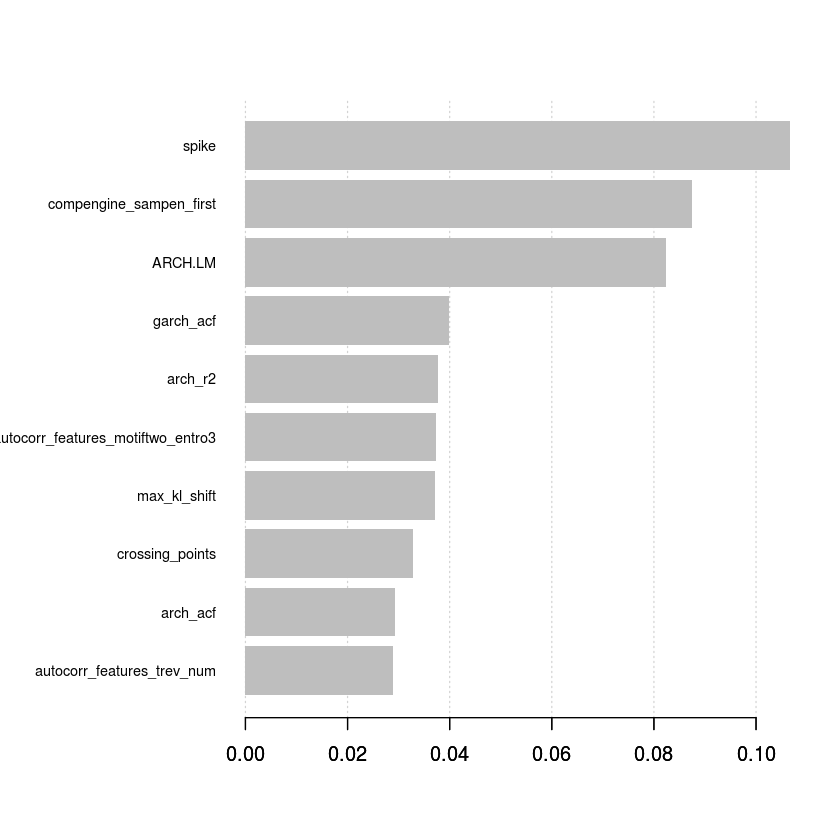In :
# I next make the predictions on the 'in-sample' held out test set, that is, originally I took the 12,000 training samples
# and split them between 75% training and 25% 'in-sample' testing (9000 training vs 3000 in-sample testing)

# I plot the probabilities from the model - the "dashed" line is the average predicted probability.
xgb.pred <- predict(xgb.model, dval, type = 'prob')

results <- cbind(y_val, xgb.pred)

results %>%
as.tibble() %>%
ggplot(aes(x = xgb.pred)) +
geom_density(color = "darkblue", fill = "lightblue") +
geom_vline(aes(xintercept = mean(xgb.pred)),
color = "blue", linetype = "dashed", size = 1) +
geom_histogram(aes(y = ..density..), colour = "black", fill = "white", alpha = 0.1, position = "identity") +
ggtitle("Predicted probability density plot") +
theme_tq()

stat_bin() using bins = 30. Pick better value with binwidth.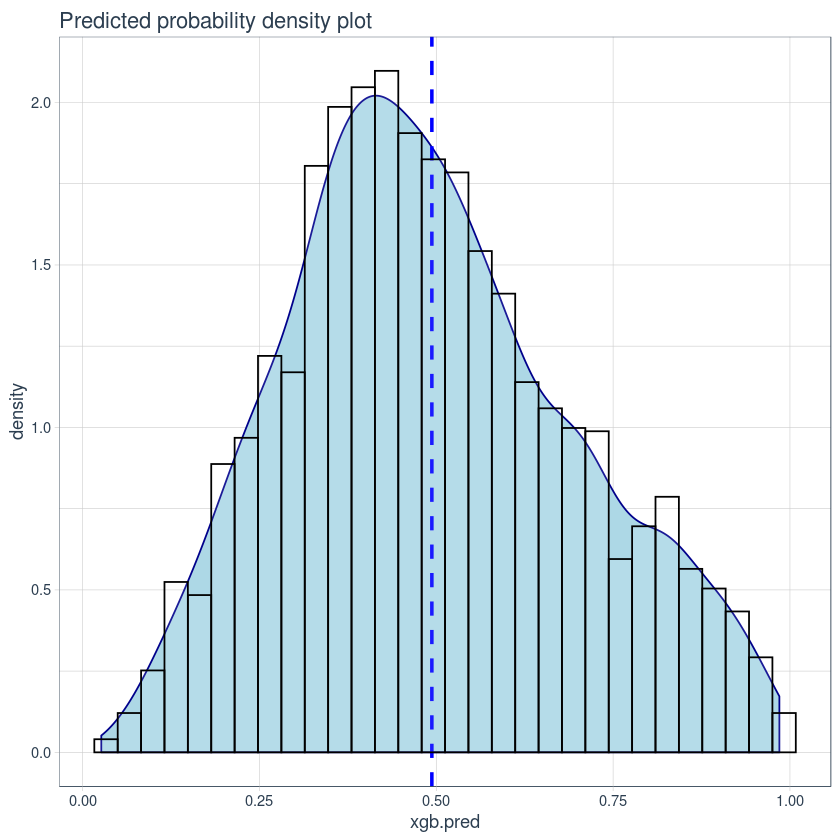In :
# The average predicted probability sits around 0.48 / 0.49, for simplicity I will just select 0.50 as the cut off threshold.
# That is, all observations <= 0.50 are assigned a "0" class or "synthetic" data and all observations >= are assigned a "1" or
# "real" data.
# Finally I output the confusion matrix on the 'in-sample' testing data.

results <- results %>%
as_tibble() %>%
mutate(pred = case_when(
xgb.pred > 0.5 ~ 1,
xgb.pred <= 0.5 ~ 0
))

confusionMatrix(factor(results$pred), factor(results$y_val))

Confusion Matrix and Statistics

Reference
Prediction    0    1
0 1103  553
1  426  918

Accuracy : 0.6737
95% CI : (0.6566, 0.6904)
No Information Rate : 0.5097
P-Value [Acc > NIR] : < 0.00000000000000022

Kappa : 0.346

Mcnemar's Test P-Value : 0.0000565

Sensitivity : 0.7214
Specificity : 0.6241
Pos Pred Value : 0.6661
Neg Pred Value : 0.6830
Prevalence : 0.5097
Detection Rate : 0.3677
Detection Prevalence : 0.5520
Balanced Accuracy : 0.6727

'Positive' Class : 0


# Part 3:¶

In :
# Not a great results but not terrible either. The model was able to predict with 67% accuracy on the data it had never seen
# before. With better feature selection (from other packages and not just the 'tsfeatures' package) the model will surely
# increase its accuracy further.

# Next I re-train the model using the whole training sample data (12,000 observations) and make the final prediction on the "test.csv"


# Create the test features¶

In :
test_final <- read.csv("test.csv") %>%
mutate(row_id = row_number()) %>%
melt(., measure.vars = 1:260) %>%
arrange(row_id)


row_idvariablevalue
1 feature1 0.033103857
1 feature2 0.008622451
1 feature3 0.004062185
1 feature4 0.008255409
1 feature5 0.055874059
1 feature6 -0.006126608
In [ ]:
functions <- ls("package:tsfeatures")[1:42]
# functions <- sample(functions, 20)

test_final <- test_final %>%
group_by(row_id) %>%
#  nest() %>%
#  sample_n(5) %>%
#  unnest() %>%
nest(-row_id) %>%
group_by(row_id) %T>%
{options(warn = -1)} %>%
summarise(Statistics = map(data, ~ data.frame(
bind_cols(
tsfeatures(.x\$value, functions))))) %>%
unnest(Statistics)

#print("Generated 106 Time Series features")

In :
# We are HERE @@@@@@@@@@@@@@@@@@@@@@@@@@@

#write.csv(test_final, "TSfeatures_test.csv")


# Load in the train and test features datasets¶

In :
# I have already created the features for the training dataset so I can just load them right back in as


A data.frame: 6 × 109
Xrow_idclassac_9_ac_9acf_features_x_acf1acf_features_x_acf10acf_features_diff1_acf1acf_features_diff1_acf10acf_features_diff2_acf1acf_features_diff2_acf10tsfeatures_entropytsfeatures_x_acf1tsfeatures_x_acf10tsfeatures_diff1_acf1tsfeatures_diff1_acf10tsfeatures_diff2_acf1tsfeatures_diff2_acf10unitroot_kpssunitroot_ppwalker_propcross
<int><int><int><dbl><dbl><dbl><dbl><dbl><dbl><dbl><dbl><dbl><dbl><dbl><dbl><dbl><dbl><dbl><dbl><dbl>
110-0.067527460 0.0097094450.05268970-0.50052990.3297018-0.67724030.61247390.9840151 0.0097094450.05268970-0.50052990.3297018-0.67724030.61247390.09938291-249.77320.5405405
220-0.042157717-0.0075901950.03874814-0.51715290.3129147-0.67278970.53793010.9864332-0.0075901950.03874814-0.51715290.3129147-0.67278970.53793010.04145992-256.04850.5019305
331 0.009959832-0.0405928740.04490357-0.50266830.3471209-0.67188850.61090060.9868568-0.0405928740.04490357-0.50266830.3471209-0.67188850.61090060.07756983-258.12950.5328185
440-0.042874797-0.0443618930.06158667-0.45714420.3184053-0.59064780.43611780.9790521-0.0443618930.06158667-0.45714420.3184053-0.59064780.43611780.21296332-262.07810.4903475
550 0.025931211-0.2447834960.14691301-0.58100730.4796508-0.67992290.62325290.9723766-0.2447834960.14691301-0.58100730.4796508-0.67992290.62325290.15063439-323.56720.5289575
660-0.076116613 0.0468555780.08583475-0.52531310.3438031-0.69015700.61307250.9806218 0.0468555780.08583475-0.52531310.3438031-0.69015700.61307250.02594138-262.34840.5250965
1. 12000
2. 109
A data.frame: 6 × 108
Xrow_idac_9_ac_9acf_features_x_acf1acf_features_x_acf10acf_features_diff1_acf1acf_features_diff1_acf10acf_features_diff2_acf1acf_features_diff2_acf10ARCH.LMtsfeatures_entropytsfeatures_x_acf1tsfeatures_x_acf10tsfeatures_diff1_acf1tsfeatures_diff1_acf10tsfeatures_diff2_acf1tsfeatures_diff2_acf10unitroot_kpssunitroot_ppwalker_propcross
<int><int><dbl><dbl><dbl><dbl><dbl><dbl><dbl><dbl><dbl><dbl><dbl><dbl><dbl><dbl><dbl><dbl><dbl><dbl>
11-0.026207279-0.0396281020.04297837-0.49642450.3379915-0.67048370.61780880.142574450.9817288-0.0396281020.04297837-0.49642450.3379915-0.67048370.61780880.88203803-252.25090.4826255
22-0.004779871 0.0544154990.04234451-0.49316530.3114689-0.69807870.65974270.111162490.9856808 0.0544154990.04234451-0.49316530.3114689-0.69807870.65974270.07222245-226.94630.5250965
33 0.037036434-0.0041962860.17812093-0.38385570.3158431-0.55350870.39483730.345020230.9627133-0.0041962860.17812093-0.38385570.3158431-0.55350870.39483730.17573108-235.07800.5598456
44-0.057602864-0.0338905650.02517169-0.49637520.2570591-0.66943370.49100060.047129570.9886539-0.0338905650.02517169-0.49637520.2570591-0.66943370.49100060.08602636-241.67520.5366795
55-0.123699426 0.0086380530.03080387-0.50253630.3330186-0.66930110.58354660.115760250.9908616 0.0086380530.03080387-0.50253630.3330186-0.66930110.58354660.11690269-266.14510.4864865
66 0.013756598-0.0889224100.06686152-0.56494360.4404459-0.70978200.71284510.075229890.9835850-0.0889224100.06686152-0.56494360.4404459-0.70978200.71284510.08699125-279.89200.5328185
1. 12000
2. 108
In :
# Here I just re-create the training and testing X features and Y features, use the optimal parameters from the cross validation
# previously and run the final training model (to make predictions on the out-of-sample test data)

x_train_final <- train_final %>%
ungroup() %>%
select(-class, -row_id, -X) %>%
as.matrix()

x_test_final <- test_final %>%
ungroup() %>%
select(-row_id, -X) %>%
as.matrix()

y_train_final <- train_final %>%
ungroup() %>%
pull(class)

dtrain_final <- xgb.DMatrix(data = as.matrix(x_train_final), label = y_train_final, missing = "NaN")
dtest_final <- xgb.DMatrix(data = as.matrix(x_test_final), missing = "NaN")

watchlist <- list("train" = dtrain_final)

params <- list("eta" = 0.1, "max_depth" = 5, "colsample_bytree" = 1, "min_child_weight" = 1, "subsample"= 1,
"objective"="binary:logistic", "gamma" = 1, "lambda" = 1, "alpha" = 0, "max_delta_step" = 0,
"colsample_bylevel" = 1, "eval_metric"= "auc",
"set.seed" = 176)

nround <- 95

xgb.model_final <- xgb.train(params, dtrain_final, nround, watchlist)
print(xgb.model_final)

	train-auc:0.708604
	train-auc:0.721700
	train-auc:0.723230
	train-auc:0.729888
	train-auc:0.735542
	train-auc:0.738081
	train-auc:0.740926
	train-auc:0.744105
	train-auc:0.746320
	train-auc:0.748644
	train-auc:0.754211
	train-auc:0.756892
	train-auc:0.761524
	train-auc:0.763882
	train-auc:0.767216
	train-auc:0.772009
	train-auc:0.772943
	train-auc:0.774261
	train-auc:0.775471
	train-auc:0.777801
	train-auc:0.780629
	train-auc:0.784384
	train-auc:0.787112
	train-auc:0.788946
	train-auc:0.791835
	train-auc:0.793142
	train-auc:0.795289
	train-auc:0.798502
	train-auc:0.799893
	train-auc:0.802186
	train-auc:0.804981
	train-auc:0.805649
	train-auc:0.807120
	train-auc:0.809020
	train-auc:0.810318
	train-auc:0.812637
	train-auc:0.814760
	train-auc:0.816024
	train-auc:0.817956
	train-auc:0.819350
	train-auc:0.821653
	train-auc:0.822729
	train-auc:0.824029
	train-auc:0.824765
	train-auc:0.826924
	train-auc:0.827804
	train-auc:0.828475
	train-auc:0.831018
	train-auc:0.832247
	train-auc:0.833265
	train-auc:0.834168
	train-auc:0.835535
	train-auc:0.836093
	train-auc:0.837008
	train-auc:0.837715
	train-auc:0.839537
	train-auc:0.840310
	train-auc:0.841701
	train-auc:0.842480
	train-auc:0.843106
	train-auc:0.844495
	train-auc:0.845348
	train-auc:0.845932
	train-auc:0.847843
	train-auc:0.849445
	train-auc:0.850345
	train-auc:0.851337
	train-auc:0.852121
	train-auc:0.852663
	train-auc:0.854132
	train-auc:0.855949
	train-auc:0.856758
	train-auc:0.857115
	train-auc:0.857954
	train-auc:0.858849
	train-auc:0.859527
	train-auc:0.859917
	train-auc:0.860590
	train-auc:0.861264
	train-auc:0.862359
	train-auc:0.863101
	train-auc:0.863794
	train-auc:0.864911
	train-auc:0.866293
	train-auc:0.866976
	train-auc:0.867436
	train-auc:0.869036
	train-auc:0.869469
	train-auc:0.869931
	train-auc:0.870681
	train-auc:0.872326
	train-auc:0.873706
	train-auc:0.875704
	train-auc:0.876178
	train-auc:0.876789
##### xgb.Booster
raw: 173.8 Kb
call:
xgb.train(params = params, data = dtrain_final, nrounds = nround,
watchlist = watchlist)
params (as set within xgb.train):
eta = "0.1", max_depth = "5", colsample_bytree = "1", min_child_weight = "1", subsample = "1", objective = "binary:logistic", gamma = "1", lambda = "1", alpha = "0", max_delta_step = "0", colsample_bylevel = "1", eval_metric = "auc", set_seed = "176", silent = "1"
xgb.attributes:
niter
callbacks:
cb.print.evaluation(period = print_every_n)
cb.evaluation.log()
# of features: 106
niter: 95
nfeatures : 106
evaluation_log:
iter train_auc
1  0.708604
2  0.721700
---
94  0.876178
95  0.876789

In :
# Make the final predictions on the 'test.csv' data and plot the probability density function.

xgb.pred_final <- predict(xgb.model_final, dtest_final, type = 'prob')

xgb.pred_final %>%
as_tibble() %>%
setNames(c("Prediction")) %>%
ggplot(aes(x = Prediction)) +
geom_density(color = "darkblue", fill = "lightblue") +
geom_vline(aes(xintercept = mean(Prediction)),
color = "blue", linetype = "dashed", size = 1) +
geom_histogram(aes(y = ..density..), colour = "black", fill = "white", alpha = 0.1, position = "identity") +
ggtitle("(Out of sample) - Predicted probability density plot") +
theme_tq()

stat_bin() using bins = 30. Pick better value with binwidth.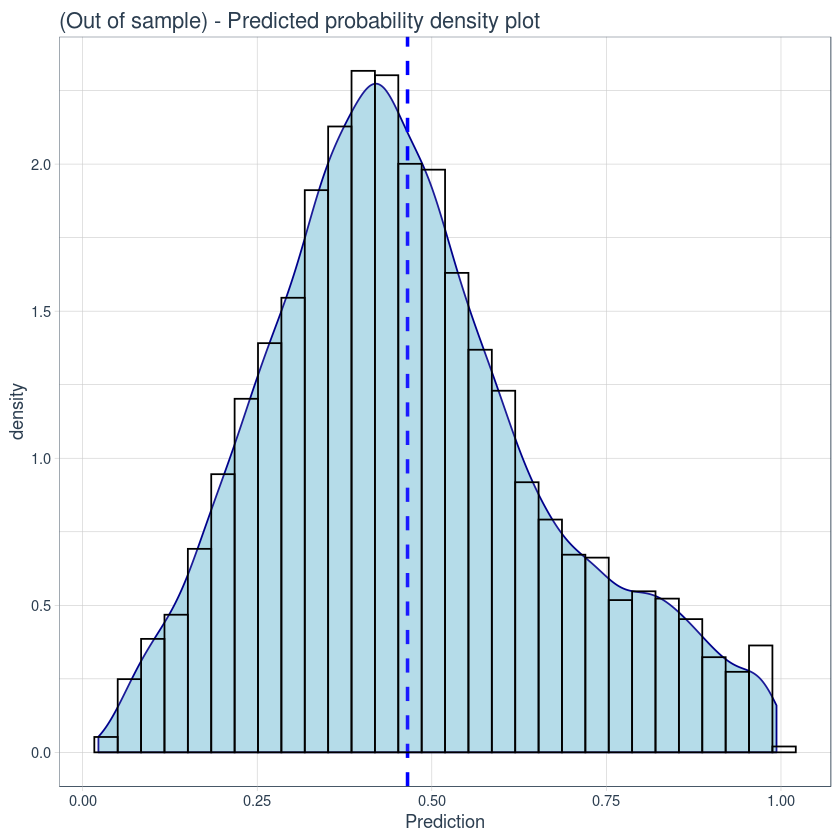In :
# Convert the probabilities into a binary class of 0 or 1 by a decision threshold of 0.465.
# Write the predictions to "submission.csv"

xgb.pred_final %>%
as_tibble() %>%
setNames(c("Prediction")) %>%
summarise(mean = mean(Prediction))

xgb.pred_final %>%
as_tibble() %>%
setNames(c("Prediction")) %>%
mutate(pred = case_when(
Prediction > 0.465 ~ 1,
Prediction <= 0.465 ~ 0
)) %>%
write.csv("submission.csv")

A tibble: 1 × 1
mean
<dbl>
0.4652583

# ------------------- END FILE -----------------¶

Final footnote: Hopefully the out-of-sample predictions will obtain a 67% accuracy (the predictions in the "submission.csv" file).